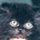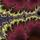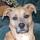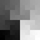## General Question# How would I reverse this equation?

Asked by frankielaguna (256) September 19th, 2010

I’m using this equation to convert steps to estimated calories lost.

I now need to do the opposite and convert total calories to estimated steps.

This is the equation I’m using for steps to calories: http://pastie.org/private/6oioyyeltnj9ogw5kq

It’s written in Objective-C, but I tried to make it as readable as possible.

Thank you for you help.

EDIT: The calculations will be over a 1 hour period.

Observing members: 0Composing members: 0## 5 AnswersWrite the function out as an algebraic formula, and solve for the intended variable. It’s very easy this way.

Vortico (3128)“Great Answer” (1) Flag as…You need to include a new variable for “Steps” (whether that is “StepsPerHour” or “StepsPerMile” depends on how you want to set up the relationships) and solve for that value over time or distance, and then multiply by total distance or time.

Either way, I expect that “StepsPerMile” would be a function of a person’s height and stride length, and “StepsPerHour” would be an indirect result of distance (miles per hour divided by steps per mile) or a direct result of “StepsPerHour” times TotalMinutesElapsed divided by 60.

Something like that.

CyanoticWasp (20072)“Great Answer” (0) Flag as…I question the accuracy of the equation. Since calories is a measure of work (in the physics sense) and time*rate= distance, it says that work = weight *distance. This would be accurate if you were climbing, that is if the distance was vertical. It gets more complicated for walking or jogging. For example, imagine that you are on a perfectly smooth surface (no friction). You could just slide at 3 miles per hour without doing any work.

LostInParadise (28466)“Great Answer” (0) Flag as…A thought occurred to me. When you are talking about steps, are you talking about a step climbing exercise? In that case, the equation would make more sense. You would still need a conversion factor from foot-pounds to Calories. A foot-pound is about .000324 Calories so I assume that is what you would use if the 3mph walking rate is given in feet per minute. On the other hand, the 3mph value may already include the conversion for Calories, in which case you can ignore the .000324 factor.

Then Calories = weight * time * rate * .000324 and
time = Calories/(weight * rate * .000324)

If you substitute steps/steps per second for time then you get
Calories = weight *steps/steps per minute * walking rate * .000324
steps = Calories*steps per minute/(walking rate * .000324)

If you want to specify both the time and the number of steps then you would have to vary the step rate and to convert to feet per minute you would have to measure the step size.

LostInParadise (28466)“Great Answer” (1) Flag as…Vortico is correct; since this is a programming problem, and not your maths homework, I’ll walk you through the algebra.

I’ll assume your original function is correct. The new function will have access to the same global variables (AverageStepsPerMinute & Walking3MphRate) and, in analogy with your function, take EstimatedCaloriesBurned and PersonsWeight as arguments.

You want to find TotalStepsTaken. This variable appears in only the first equation:

(1) TotalMinutesElapsed = TotalStepsTaken / AverageStepsPerMinute

To solve for it, multiply both sides by AverageStepsPerMinute:

(2) TotalMinutesElapsed * AverageStepsPerMinute = TotalStepsTaken

But you don’t know the value of TotalMinutesElapsed. It also occurs in your second equation:

(3) EstimatedCaloriesBurned = PersonsWeight * TotalMinutesElapsed * Walking3MphRate

So we solve for it by dividing both sides by PersonsWeight * Walking3MphRate:

(4) EstimatedCaloriesBurned / (PersonsWeight * Walking3MphRate) = TotalMinutesElapsed

Switch the order of the two solutions (equations (2) and (4)) and convert them into code (don’t forget to swap the left- and right-hand sides of the equality signs), and you have your new function. (This is left as an exercise for the reader.)

P.S.: By substituting equation (4) into (2), you can write more tersely

(5) TotalStepsTaken = EstimatedCaloriesBurned * AverageStepsPerMinute / (PersonsWeight * Walking3MphRate)

But I think your method of using two equations makes it clearer to read and debug.

Dan337 (177)“Great Answer” (0) Flag as…## Answer this questionor

#### Join

to answer.

This question is in the General Section. Responses must be helpful and on-topic.

Your answer will be saved while you login or join.
Have a question? Ask Fluther!
What do you know more about?
or
Knowledge Networking @ Fluther